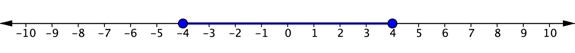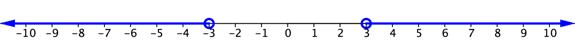## 6.3 – Equations and Inequalities with Absolute Value

### Learning Objectives

• (6.3.1) – Solve equations containing absolute values
• Equations with one absolute value
• Equations with two absolute values
• Recognize when a linear equation that contains absolute value does not have a solution
• (6.3.2) – Solve inequalities containing absolute values

# (6.3.1) – Solve equations containing absolute values

Next, we will learn how to solve an absolute value equation. To solve an equation such as $|2x - 6|=8$, we notice that the absolute value will be equal to 8 if the quantity inside the absolute value bars is $8$ or $-8$. This leads to two different equations we can solve independently.

$\begin{array}{lll}2x - 6=8\hfill & \text{ or }\hfill & 2x - 6=-8\hfill \\ 2x=14\hfill & \hfill & 2x=-2\hfill \\ x=7\hfill & \hfill & x=-1\hfill \end{array}$

Knowing how to solve problems involving absolute value functions is useful. For example, we may need to identify numbers or points on a line that are at a specified distance from a given reference point.

### A General Note: Absolute Value Equations

The absolute value of x is written as $|x|$. It has the following properties:

$\begin{array}{l}\text{If } x\ge 0,\text{ then }|x|=x.\hfill \\ \text{If }x<0,\text{ then }|x|=-x.\hfill \end{array}$

For real numbers $A$ and $B$, an equation of the form $|A|=B$, with $B\ge 0$, will have solutions when $A=B$ or $A=-B$. If $B<0$, the equation $|A|=B$ has no solution.

An absolute value equation in the form $|ax+b|=c$ has the following properties:

$\begin{array}{l}\text{If }c<0,|ax+b|=c\text{ has no solution}.\hfill \\ \text{If }c=0,|ax+b|=c\text{ has one solution}.\hfill \\ \text{If }c>0,|ax+b|=c\text{ has two solutions}.\hfill \end{array}$

### How To: Given an absolute value equation, solve it.

1. Isolate the absolute value expression on one side of the equal sign.
2. If $c>0$, write and solve two equations: $ax+b=c$ and $ax+b=-c$.

In the next video, we show examples of solving a simple absolute value equation.

### Example: Solving Absolute Value Equations

Solve the following absolute value equations:

1. $|6x+4|=8$
2. $|3x+4|=-9$
3. $|3x - 5|-4=6$
4. $|-5x+10|=0$

In the two videos that follow, we show examples of how to solve an absolute value equation that requires you to isolate the absolute value first using mathematical operations.

### Try It

Solve the absolute value equation: $|1 - 4x|+8=13$.

### Equations with two absolute values

Some of our absolute value equations could be of the form $|u|=|v|$ where $u$ and $v$ are algebraic expressions. For example, $|x-3|=|2x+1|$.

How would we solve them? If two algebraic expressions are equal in absolute value, then they are either equal to each other or negatives of each other. The property for absolute value equations says that for any algebraic expression, $u$, and a positive real number, $a$, if $|u|=a$, then $u=a$ or $u=-a$.

This leads us to the following property for equations with two absolute values:

### Equations with Two Absolute Values

For any algebraic expressions, $u$ and $v$, if $|u|=|v|$, then:

$u=v$ or $u=-v$.

When we take the opposite of a quantity, we must be careful with the signs and to add parentheses where needed.

### ExAMPLE

Solve: $|5x-1|=|2x+3|$.

### Absolute value equations with no solutions

As we are solving absolute value equations it is important to be aware of special cases. An absolute value is defined as the distance from 0 on a number line, so it must be a positive number. When an absolute value expression is equal to a negative number, we say the equation has no solution, or DNE. Notice how this happens in the next two examples.

### Example

Solve for $x$. $7+\left|2x-5\right|=4$

### Example

Solve for $x$. $-\frac{1}{2}\left|x+3\right|=6$

In this last video, we show more examples of absolute value equations that have no solutions.

# (6.3.2) – Solve inequalities containing absolute values

Let’s apply what you know about solving equations that contain absolute values and what you know about inequalities to solve inequalities that contain absolute values. Let’s start with a simple inequality.

$\left|x\right|\leq 4$

This inequality is read, “the absolute value of $x$ is less than or equal to 4.” If you are asked to solve for $x$, you want to find out what values of $x$ are 4 units or less away from 0 on a number line. You could start by thinking about the number line and what values of $x$ would satisfy this equation.

4 and $−4$ are both four units away from 0, so they are solutions. 3 and $−3$ are also solutions because each of these values is less than 4 units away from 0. So are 1 and $−1$, 0.5 and $−0.5$, and so on—there are an infinite number of values for $x$ that will satisfy this inequality.

The graph of this inequality will have two closed circles, at 4 and $−4$. The distance between these two values on the number line is colored in blue because all of these values satisfy the inequality.The solution can be written this way:

Inequality: $-4\leq x\leq4$

Interval: $\left[-4,4\right]$

The situation is a little different when the inequality sign is “greater than” or “greater than or equal to.” Consider the simple inequality $\left|x\right|>3$. Again, you could think of the number line and what values of $x$ are greater than 3 units away from zero. This time, 3 and $−3$ are not included in the solution, so there are open circles on both of these values. 2 and $−2$ would not be solutions because they are not more than 3 units away from 0. But 5 and $−5$ would work, and so would all of the values extending to the left of $−3$ and to the right of 3. The graph would look like the one below.The solution to this inequality can be written this way:

Inequality: $x<−3$ or $x>3$.

Interval: $\left(-\infty, -3\right)\cup\left(3,\infty\right)$

In the following video, you will see examples of how to solve and express the solution to absolute value inequalities involving both AND and OR.

#### Writing Solutions to Absolute Value Inequalities

For any positive value of $a$ and $x$, a single variable, or any algebraic expression:

 Absolute Value Inequality Equivalent Inequality Interval Notation $\left|{ x }\right|\le{ a}$ ${ -a}\le{x}\le{ a}$ $\left[-a, a\right]$ $\left| x \right|\lt{a}$ ${ -a}\lt{x}\lt{ a}$ $\left(-a, a\right)$ $\left| x \right|\ge{ a}$ ${x}\le\text{−a}$ or ${x}\ge{ a}$ $\left(-\infty,-a\right]\cup\left[a,\infty\right)$ $\left| x \right|\gt\text{a}$ $\displaystyle{x}\lt\text{−a}$ or ${x}\gt{ a}$ $\left(-\infty,-a\right)\cup\left(a,\infty\right)$

Let’s look at a few more examples of inequalities containing absolute values.

### Example

Solve for $x$. $\left|x+3\right|\gt4$

### Example

Solve for $y$$\displaystyle 3\left|2y+6\right|-9<27$

In the following video, you will see an example of solving multi-step absolute value inequalities involving an OR situation.

In the following video you will see an example of solving multi-step absolute value inequalities involving an AND situation.

In the last video that follows, you will see an example of solving an absolute value inequality where you need to isolate the absolute value first.

#### Identify cases of inequalities containing absolute values that have no solutions

As with equations, there may be instances in which there is no solution to an inequality.

### Example

Solve for $x$. $\left|2x+3\right|+9\leq 7$

#### Summary

Absolute inequalities can be solved by rewriting them using compound inequalities. The first step to solving absolute inequalities is to isolate the absolute value. The next step is to decide whether you are working with an OR inequality or an AND inequality. If the inequality is greater than a number, we will use OR. If the inequality is less than a number, we will use AND. Remember that if we end up with an absolute value greater than or less than a negative number, there is no solution.